# 游戏演示

2048小游戏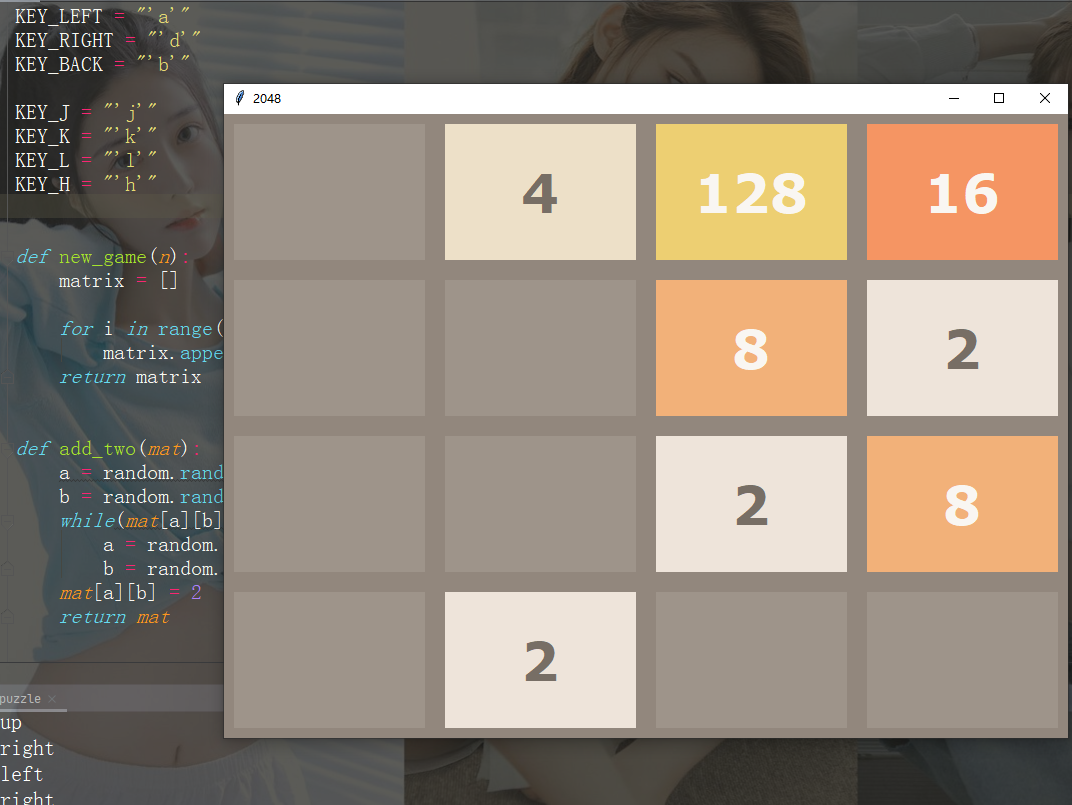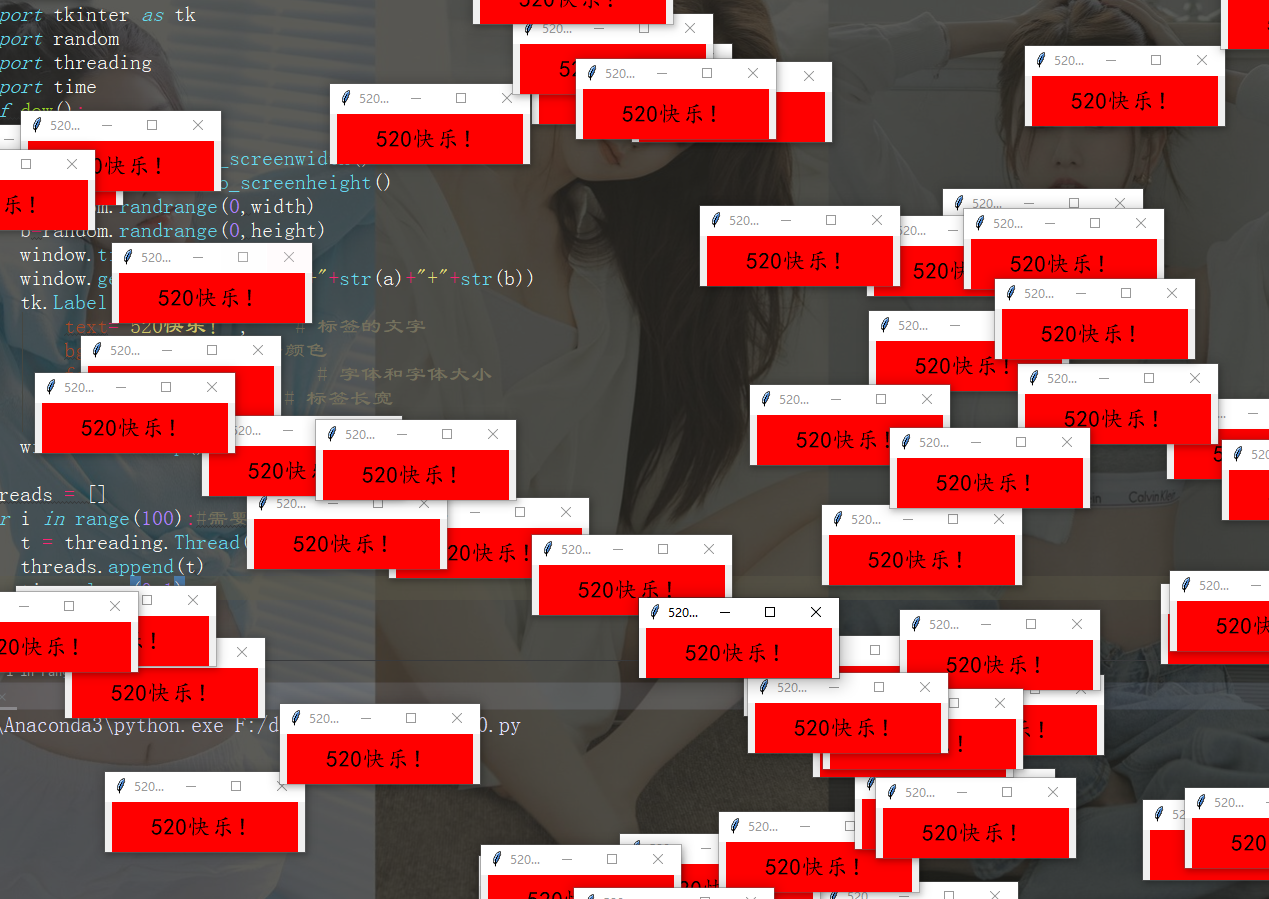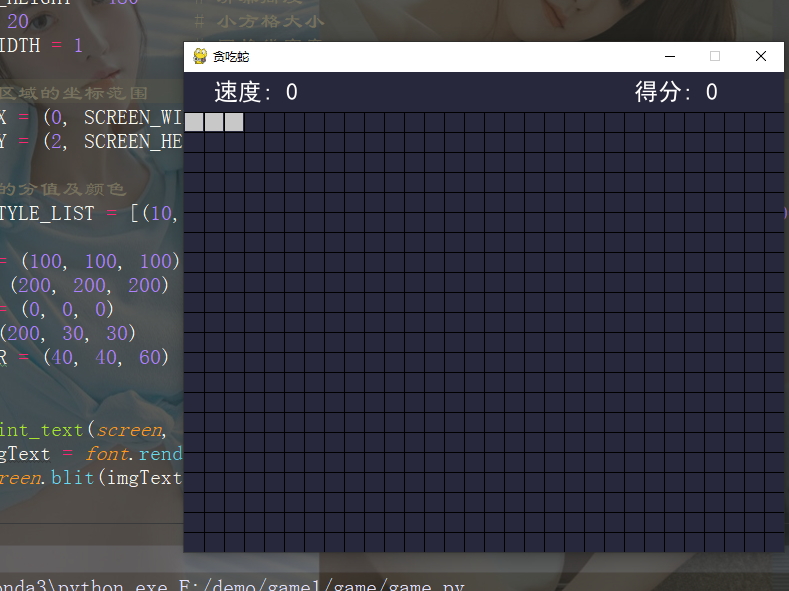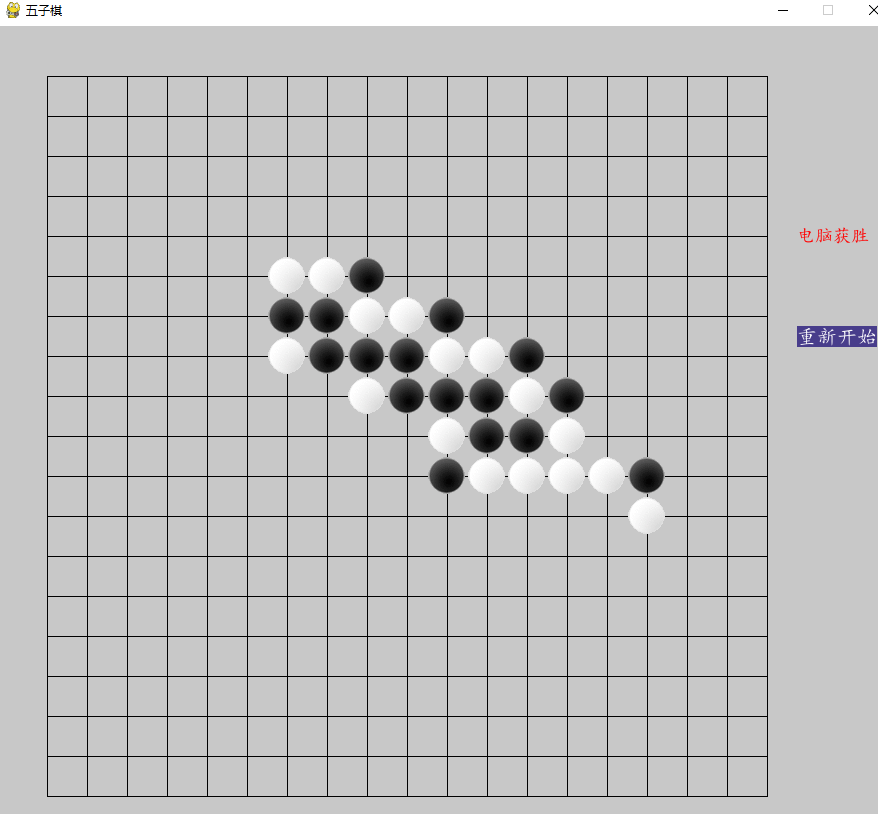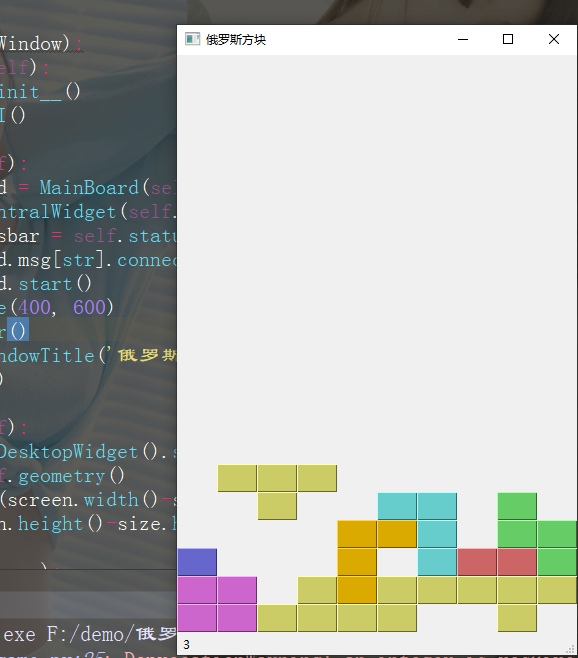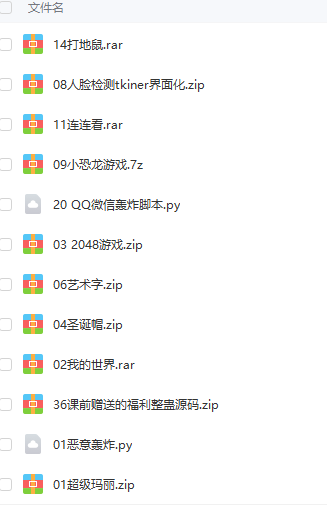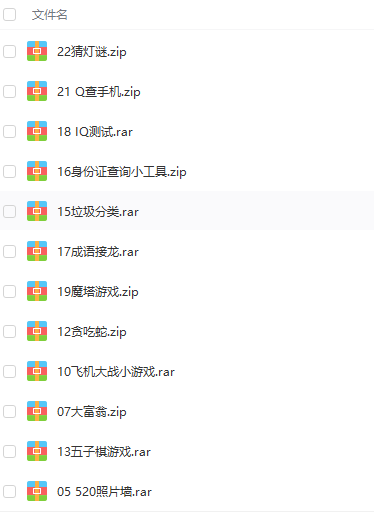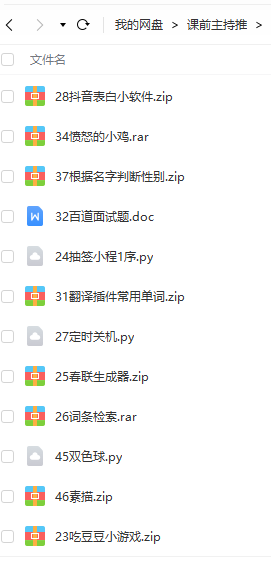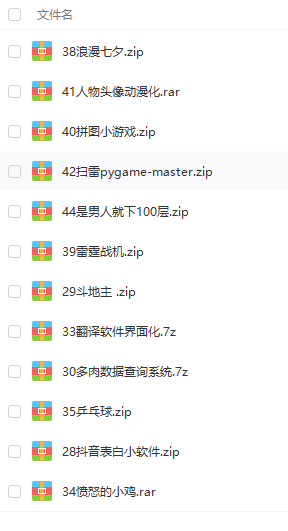# 基本框架

```import pygame
# 以上游戏源码可以加群279199867或者 WeChat ：Python10010 获取哦

def main():
pygame.init()
pygame.display.set_caption('未闻Code：青南做的游戏')  # 游戏标题
win = pygame.display.set_mode((800, 600))  # 窗口尺寸，宽800高600
running = True
while running:
for event in pygame.event.get():
if event.type == pygame.QUIT:  # 点击左上角或者右上角的x关闭窗口时，停止程序
running = False

main()```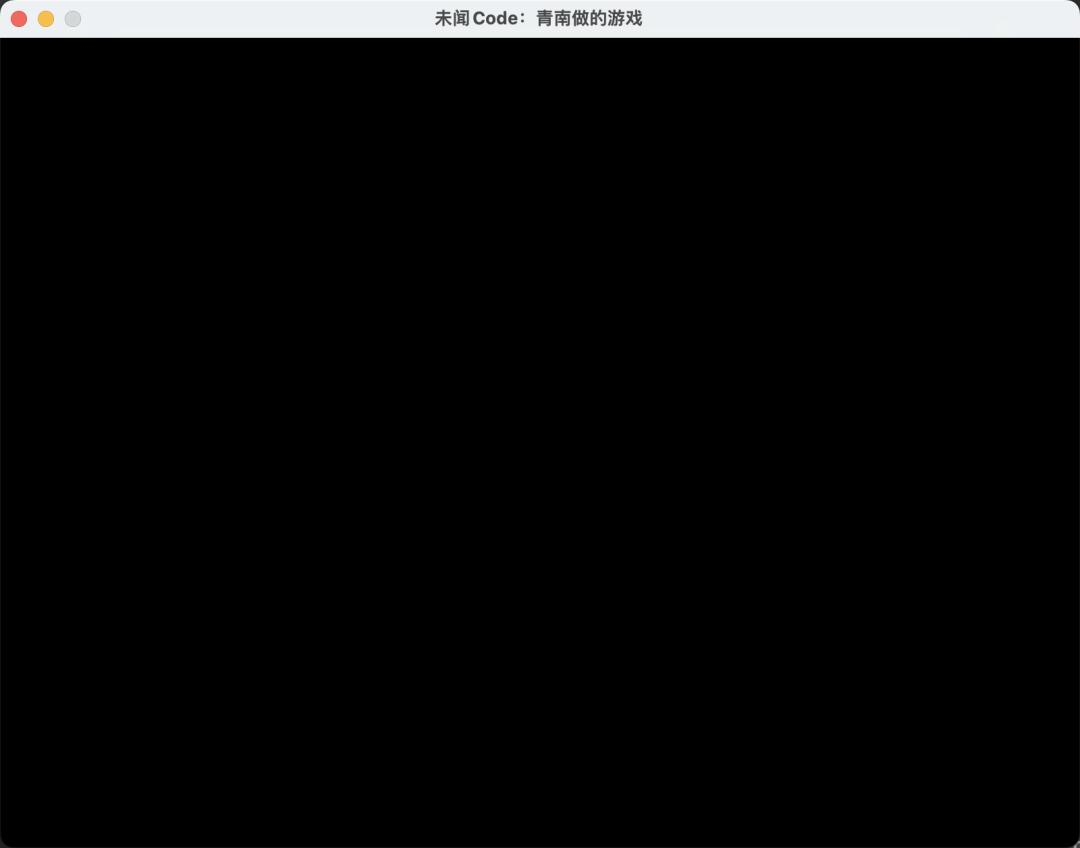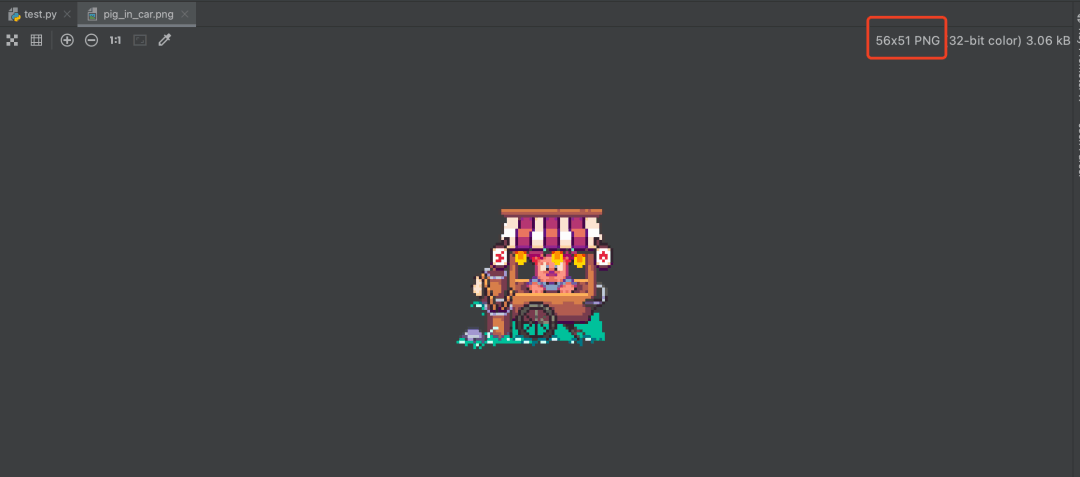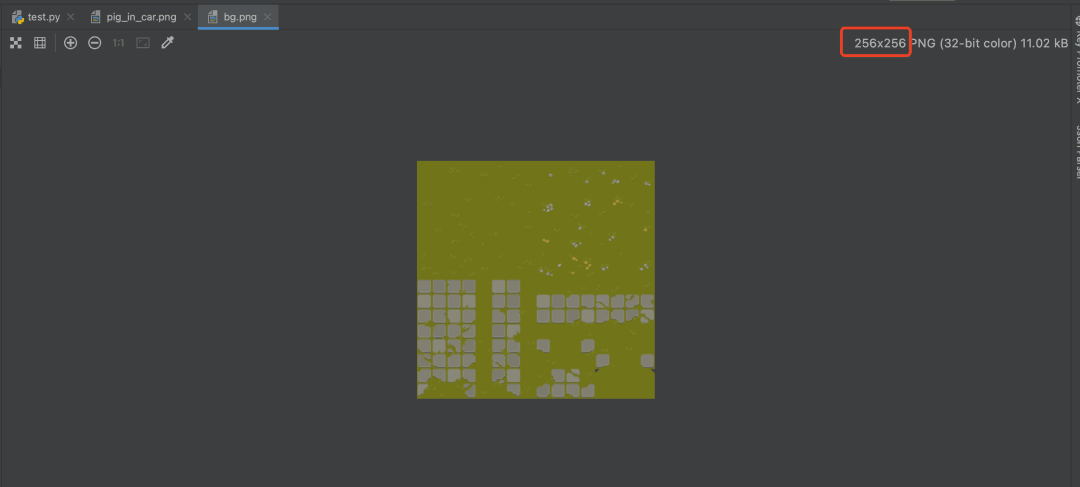`img_surf = pygame.image.load('图片地址').convert_alpha()`

`img_surf = pygame.transform.scale(img_surf, (宽, 高))`

```win.blit(素材对象, (素材左上角的横坐标, 素材左上角的纵坐标))
pygame.display.flip()```

```import pygame

def main():
pygame.init()
pygame.display.set_caption('未闻Code：青南做的游戏')  # 游戏标题
win = pygame.display.set_mode((800, 600))  # 窗口尺寸
bg_big = pygame.transform.scale(bg_small, (800, 600))
running = True
while running:
for event in pygame.event.get():
if event.type == pygame.QUIT:  # 点击左上角或者右上角的x关闭窗口时，停止程序
running = False

win.blit(bg_big, (0, 0))  # 背景图最先加载，坐标是(left, top)
win.blit(pig, (200, 300))
pygame.display.flip()

main()```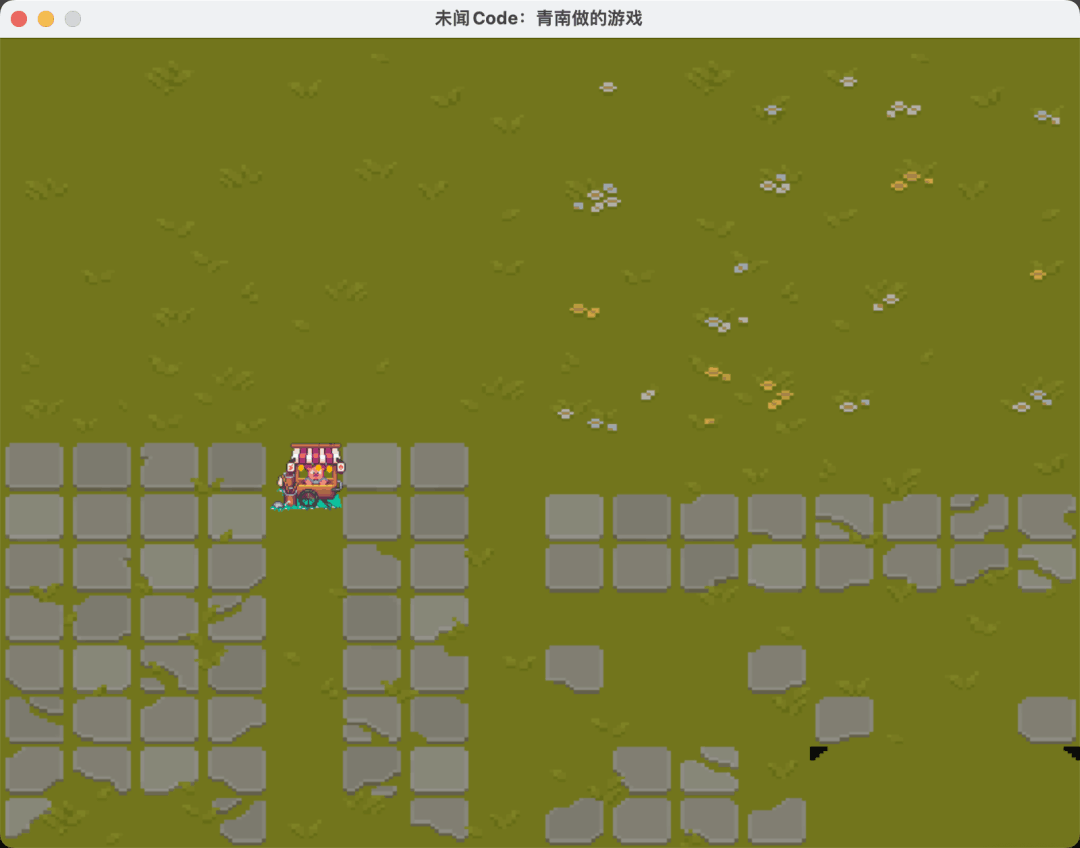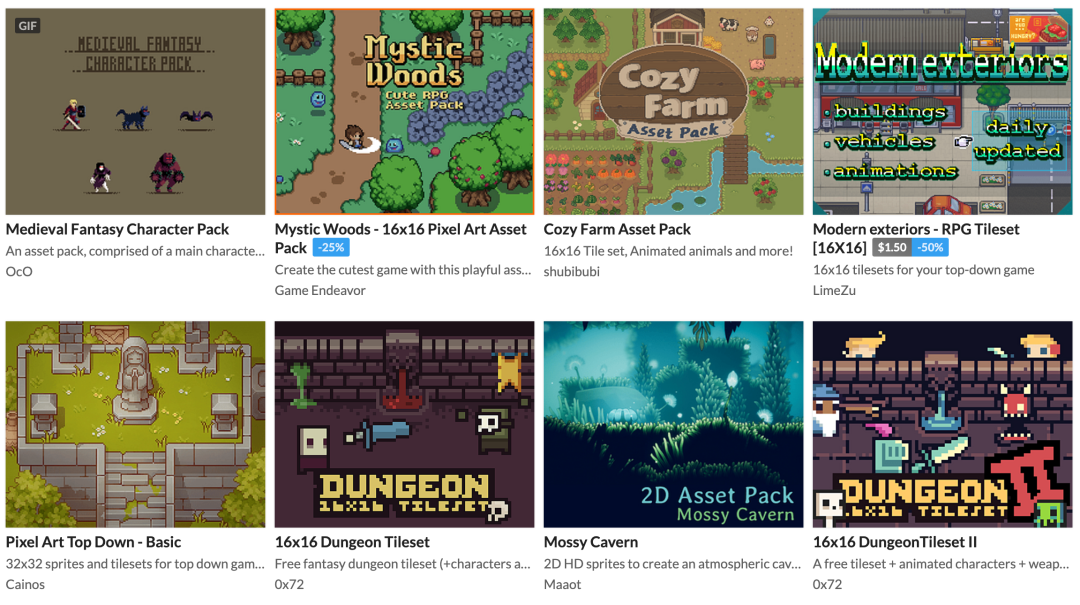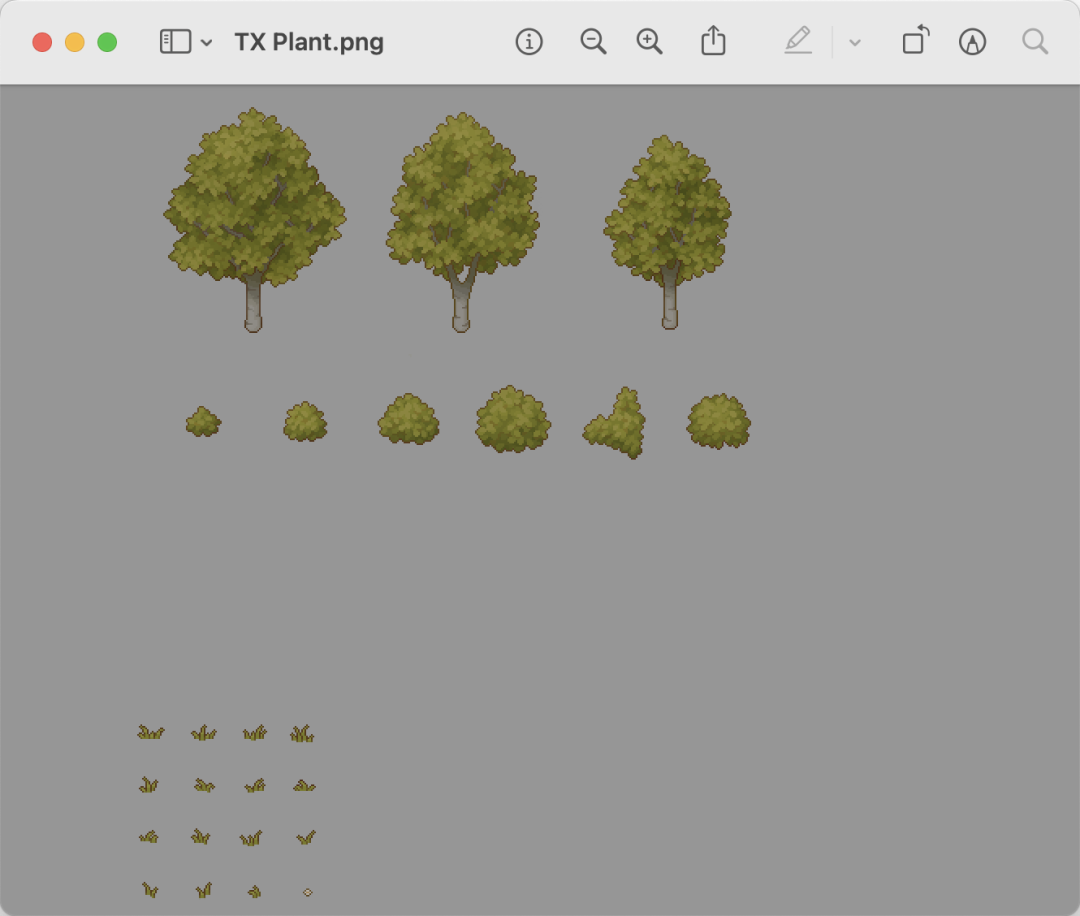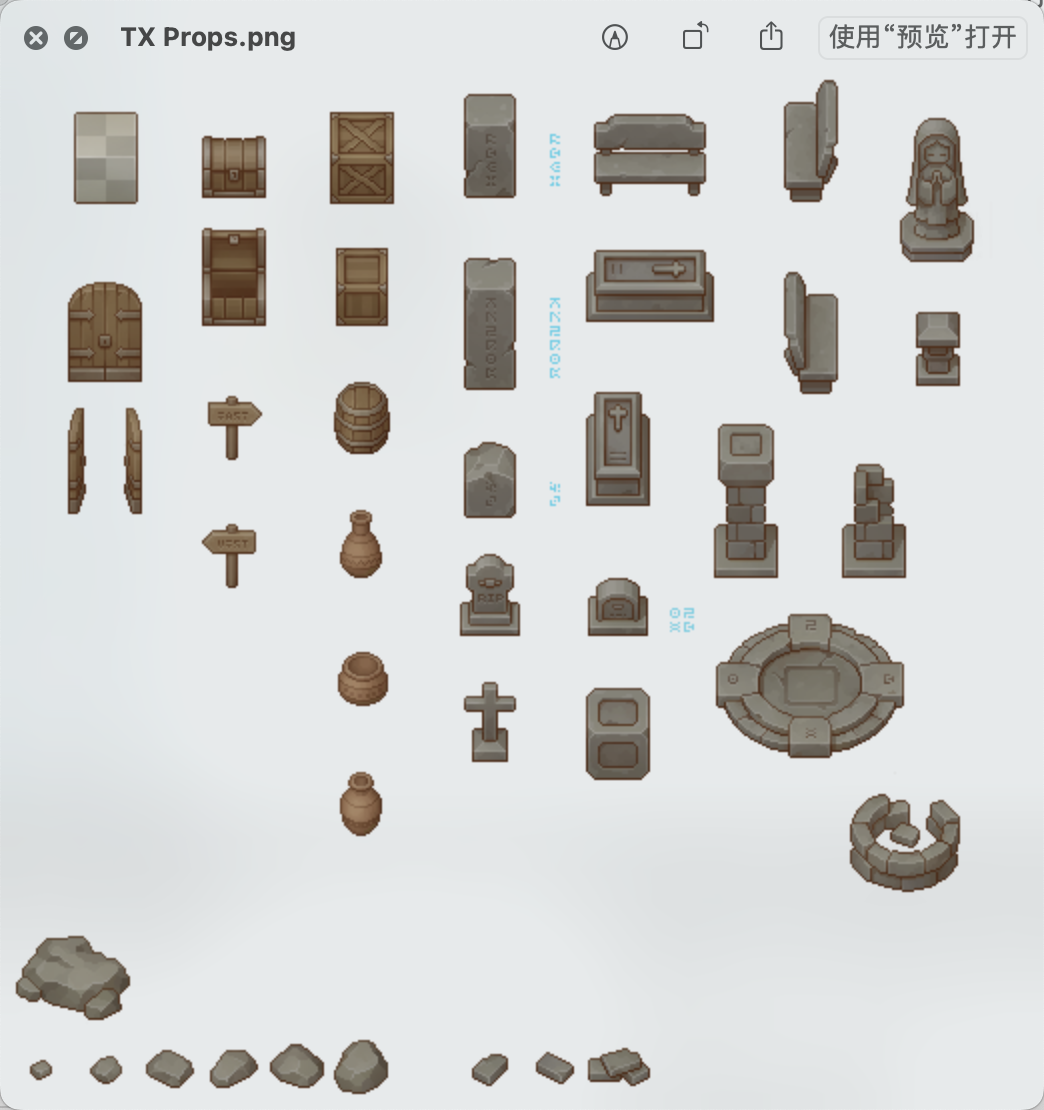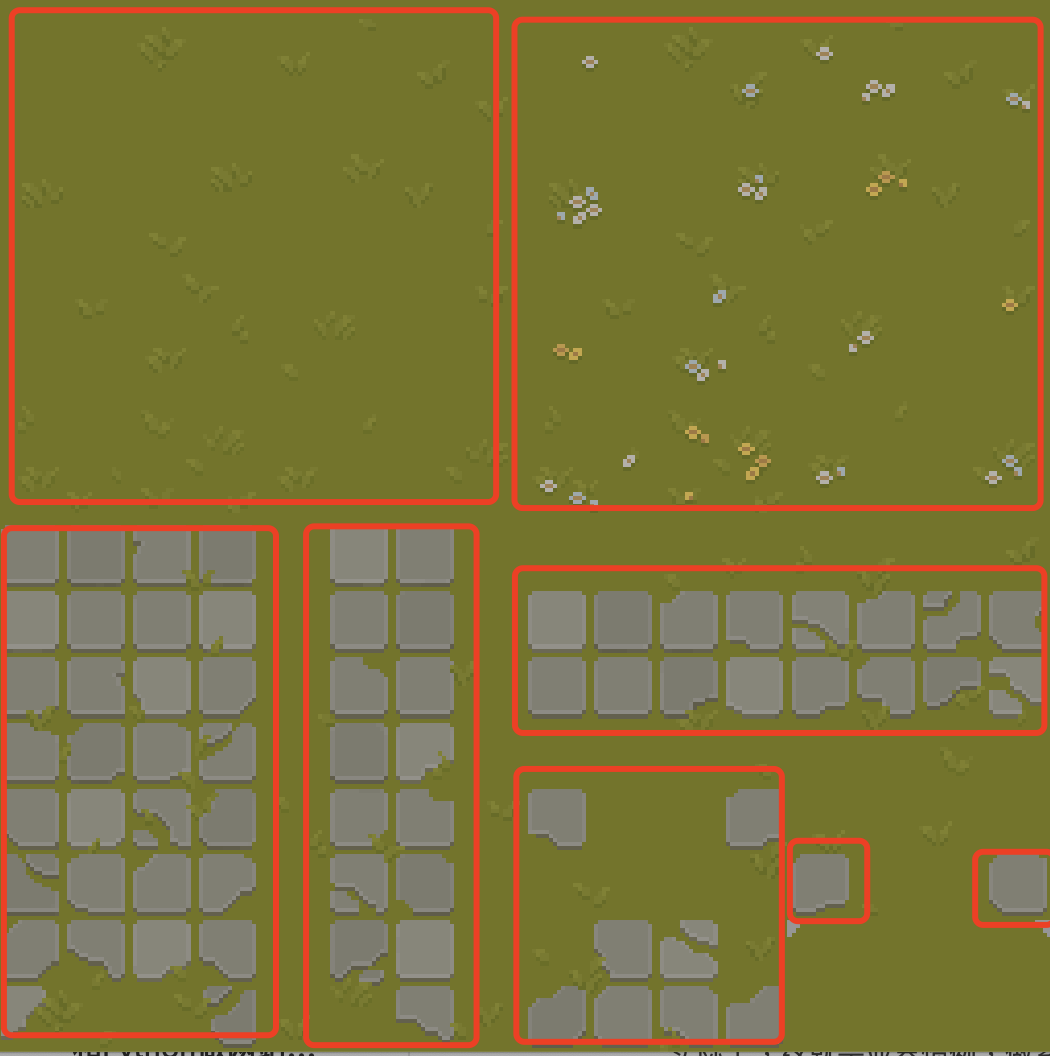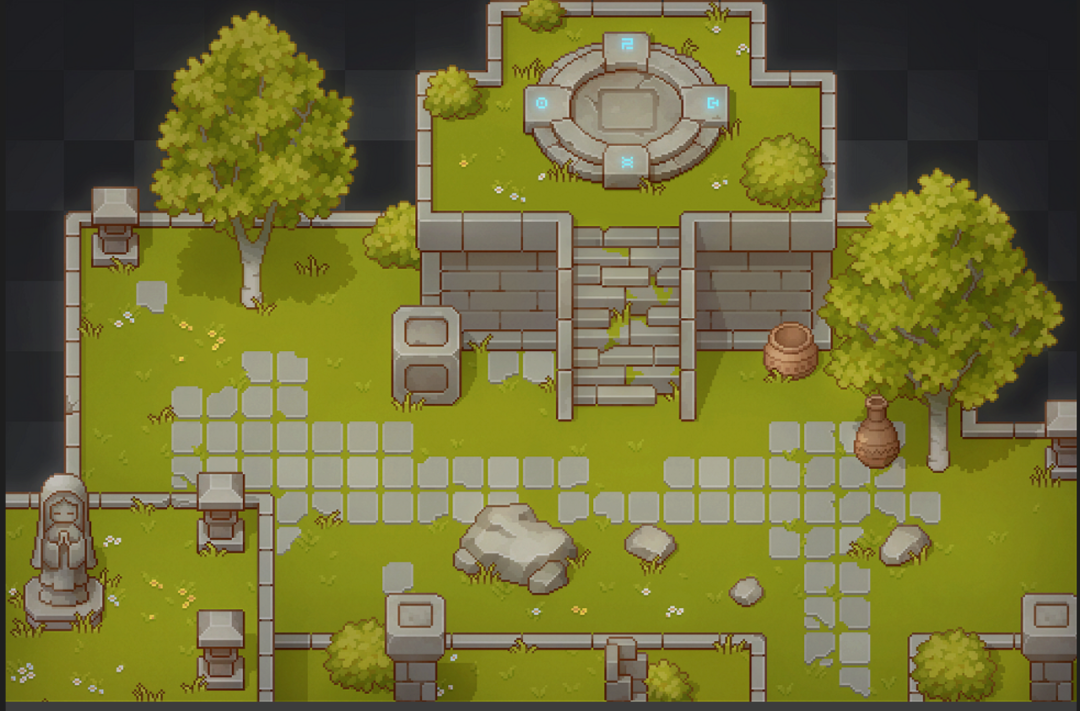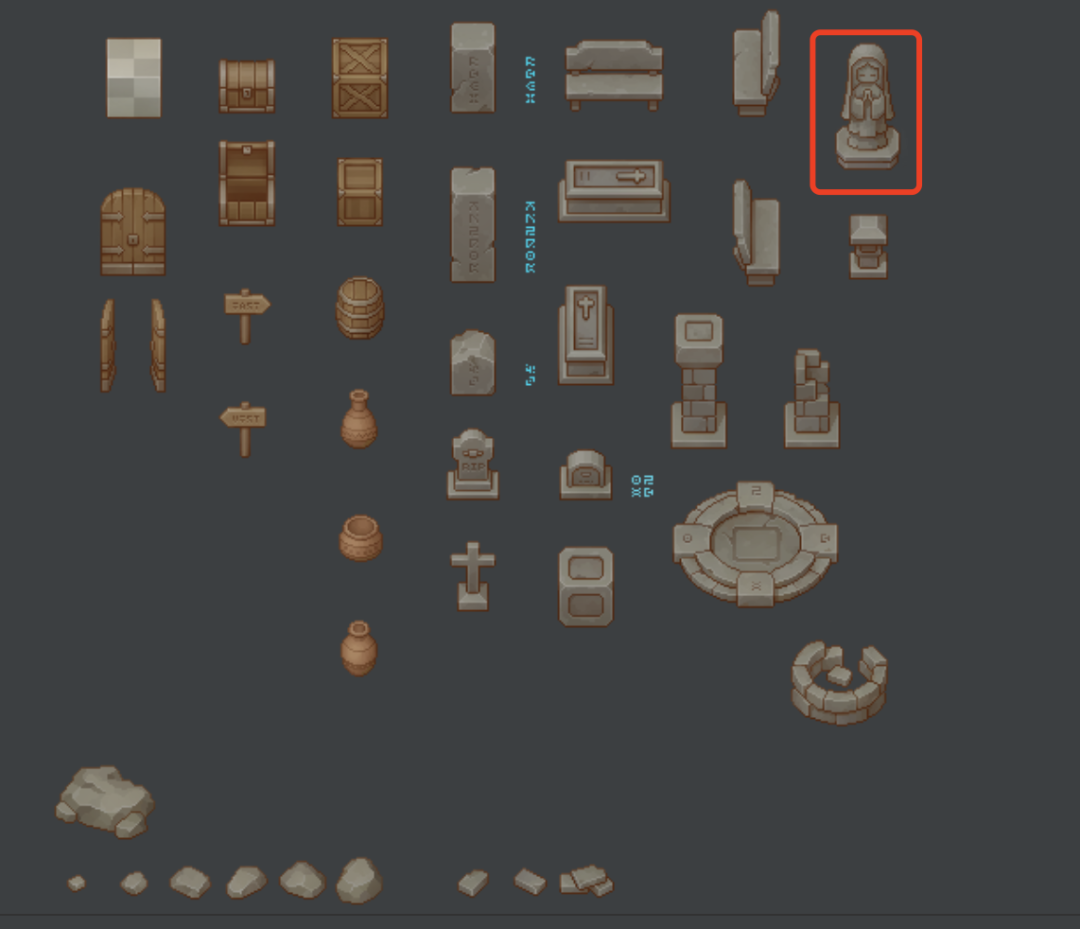```img_surf = pygame.image.load('雕像素材.png').convert_alpha()
goddess= img_surf.subsurface(( 女神像左上角的横坐标 , 女神像左上角的纵坐标, 女神像的宽, 女神像的高))```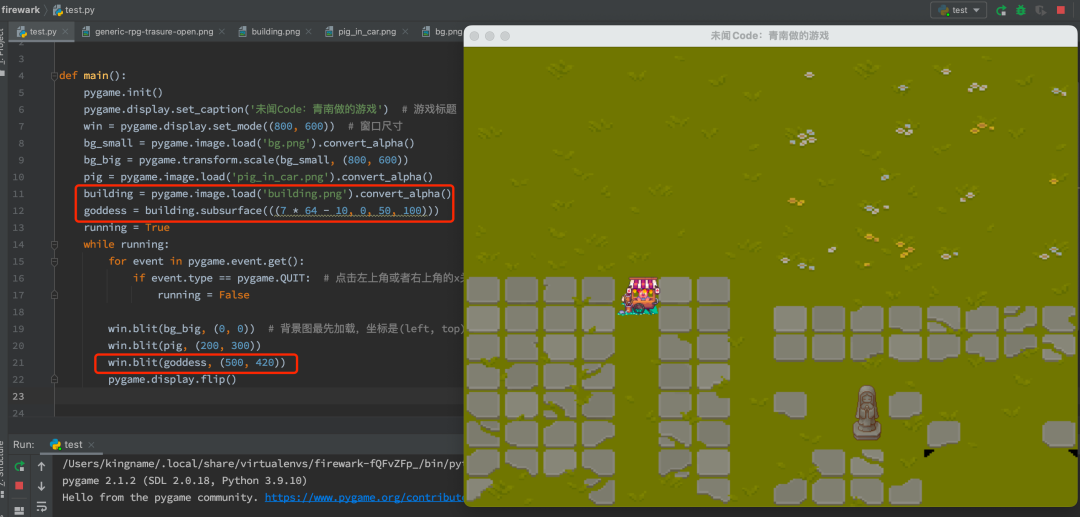```import pygame

class Bg(pygame.sprite.Sprite):
def __init__(self):
super(Bg, self).__init__()
grass_land = bg_small.subsurface((0, 0, 128, 128))
self.surf = pygame.transform.scale(grass_land, (800, 600))
self.rect = self.surf.get_rect(left=0, top=0)  # 左上角定位

class Pig(pygame.sprite.Sprite):
def __init__(self):
super(Pig, self).__init__()
self.rect = self.surf.get_rect(center=(400, 300))  # 中心定位

class Goddess(pygame.sprite.Sprite):
def __init__(self):
super(Goddess, self).__init__()
self.surf = building.subsurface(((7 * 64 - 10, 0, 50, 100)))
self.rect = self.surf.get_rect(center=(500, 430))  # 女神像的中心放到画布(500, 430)的位置

def main():
pygame.init()
pygame.display.set_caption('未闻Code：青南做的游戏')  # 游戏标题
win = pygame.display.set_mode((800, 600))  # 窗口尺寸

bg = Bg()
goddess = Goddess()
pig = Pig()
all_sprites = [bg, goddess, pig]  # 注意添加顺序，后添加的对象图层在先添加的对象的图层上面

running = True
while running:
for event in pygame.event.get():
if event.type == pygame.QUIT:  # 点击左上角或者右上角的x关闭窗口时，停止程序
running = False

for sprite in all_sprites:
win.blit(sprite.surf, sprite.rect)
pygame.display.flip()

if __name__ == '__main__':
main()```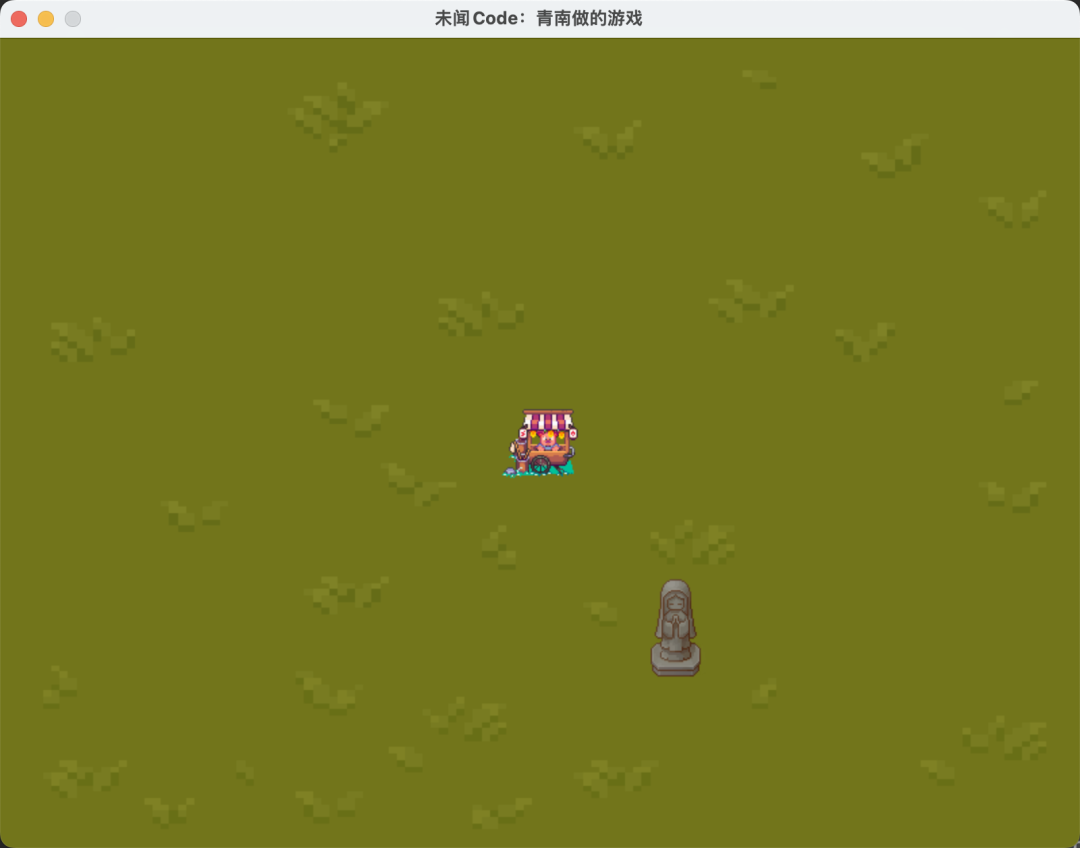PyGame 本质上，就是通过win.blit不停地画图，由于这个while循环每秒要运行很多次，如果每次运行的时候，我们让win.blit的第二个参数，也就是素材对象的坐标有细微的差异，那么在人眼看起来，这个素材对象就在运动了。

`keys = pygame.key.get_pressed()`

```class Pig(pygame.sprite.Sprite):
def __init__(self):
super(Pig, self).__init__()
self.rect = self.surf.get_rect(center=(400, 300))  # 中心定位

def update(self, keys):
if keys[pygame.K_LEFT]:
self.rect.move_ip((-5, 0))  # 横坐标向左
elif keys[pygame.K_RIGHT]:
self.rect.move_ip((5, 0))  # 横坐标向右
elif keys[pygame.K_UP]:
self.rect.move_ip((0, -5))  #纵坐标向上
elif keys[pygame.K_DOWN]:
self.rect.move_ip((0, 5))  # 纵坐标向下

# 防止小猪跑到屏幕外面
if self.rect.left < 0:
self.rect.left = 0
if self.rect.right > 800:
self.rect.right = 800
if self.rect.top < 0:
self.rect.top = 0
if self.rect.bottom > 600:
self.rect.bottom = 600```

.update方法接收一个参数keys，就是我们按键返回的长得像列表的对象。然后判断是哪个方向键被按下了。根据被按下的键，.rect坐标定位对象修改相应方向的值。rect.move_ip这里的ip是inplace的简写，也就是修改.rect这个属性自身。它的参数是一个元组，对应横坐标和纵坐标。横纵坐标小于 0 表示向左或者向上，大于 0 表示向右或者向下。

```keys = pygame.key.get_pressed()
pig.update(keys)```

```import pygame

class Bg(pygame.sprite.Sprite):
def __init__(self):
super(Bg, self).__init__()
grass_land = bg_small.subsurface((0, 0, 128, 128))
self.surf = pygame.transform.scale(grass_land, (800, 600))
self.rect = self.surf.get_rect(left=0, top=0)  # 左上角定位

class Pig(pygame.sprite.Sprite):
def __init__(self):
super(Pig, self).__init__()
self.rect = self.surf.get_rect(center=(400, 300))  # 中心定位

def update(self, keys):
if keys[pygame.K_LEFT]:
self.rect.move_ip((-5, 0))
elif keys[pygame.K_RIGHT]:
self.rect.move_ip((5, 0))
elif keys[pygame.K_UP]:
self.rect.move_ip((0, -5))
elif keys[pygame.K_DOWN]:
self.rect.move_ip((0, 5))

# 防止小猪跑到屏幕外面
if self.rect.left < 0:
self.rect.left = 0
if self.rect.right > 800:
self.rect.right = 800
if self.rect.top < 0:
self.rect.top = 0
if self.rect.bottom > 600:
self.rect.bottom = 600

class Goddess(pygame.sprite.Sprite):
def __init__(self):
super(Goddess, self).__init__()
self.surf = building.subsurface(((7 * 64 - 10, 0, 50, 100)))
self.rect = self.surf.get_rect(center=(500, 430))  # 女神像的中心放到画布(500, 430)的位置

def main():
pygame.init()
pygame.display.set_caption('未闻Code：青南做的游戏')  # 游戏标题
win = pygame.display.set_mode((800, 600))  # 窗口尺寸

bg = Bg()
goddess = Goddess()
pig = Pig()
all_sprites = [bg, goddess, pig]  # 注意添加顺序，后添加的对象图层在先添加的对象的图层上面

running = True
while running:
for event in pygame.event.get():
if event.type == pygame.QUIT:  # 点击左上角或者右上角的x关闭窗口时，停止程序
running = False

keys = pygame.key.get_pressed()
pig.update(keys)
for sprite in all_sprites:
win.blit(sprite.surf, sprite.rect)
pygame.display.flip()

if __name__ == '__main__':
main()```# 总结

PyGame 做游戏真的非常简单，只要会加载素材，就能做出一个还能看得过去的游戏。今天我们学会了怎么添加素材，怎么捕获键盘事件。

PyGame 可以读取 Gif 图片，但是你会发现加载进来以后，Gif 不会动。下一篇文章，我们来讲讲如何让你控制的角色动起来，例如控制一个小娃娃，移动的时候，它的脚也跟着动。以及对象的碰撞检测。

Python爬虫入门到实战100集

python tkinter实战合集